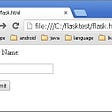# Linear Regressions — The Basic

## How to Understand Linear Regression Once and For All! — #PySeries#Episode 04

Two variables can be related to each other.

For example, we can predict the number of likes a trending YouTube video gets based on the number of comments that it has.

Experience tells us that the greater the number of comments, the greater the number of likes YouTube video gets. Is that really like this? But how?

How to describe these relationships between variables?

Today we’ll introduce you to one of the most flexible statistical tools — the Generalized Linear Model, or GLM. Here is the formula:

`Yi = β0 + β1xi + Ɛ`

Where:

`Yi - model the expected value of a continuous variableβ0 - parameter estimates the interceptβ1 - parameter estimates of the slopexi - parameter observations Ɛ  - errors(You may want to refer to the Statistics Symbol Sheet)`

The GLM will allow us to create many different models to help describe the world (Linear Regression, ANOVA, Logistic, Binomial, Poisson, etc).

The first we’ll talk about is The Regression Model.

Let’s suppose my model is this:

`Predict Likes = Likes if Zero Comments + M x Increases in Likes per comments`

Which can be translated to:

`Likes = 9.104 + 6.4954 x Number of Comments`

That is my Model!

What is the predictive strength of this model?

So, according to my Model, the number of Likes would be:

`Likes = 9.104 + (6.4954 x 10)Likes = 74.058`

Let’s suppose again that, in reality, I’ve got just 50 Likes out of 10 comments.

This is the ERROR of the Model:

`74 = 50 + ERROR(e)74 = 50 + 24`

As you know, reality doesn’t always match predictions!

Now, the error doesn’t mean that something’s WRONG, per se.

We call it an error because it’s a deviation from our model.

So the data isn’t wrong, the model is!

And these errors can come from many sources: like variables, we didn’t account for in our model — including some characteristic inherent to my English accent, since I am not native— or just random variation.

Models allow us to make inferences.

The inference is using observation and background to reach a logical conclusion.

You probably practice inference every day. For example, if you see someone eating a portion of new food and he or she makes a face, then you infer he does not like it. Or if someone slams a door, you can infer that she is upset about something.

Generalized Linear Models take the information that data give us and portion it out into two major parts: information that can be accounted for by our model, and information that can’t be.

There are many types of GLMS, one is Linear Regression, which can also provide a prediction for our data.

It uses continuous data. Here is the formula:

What is a Linear Regression after all?

The general goal of regression is to develop an equation that best describes the relationship between variables.

A regression line is a straight line that’s as close as possible to all the data points at once.

That means that it’s the one straight line that minimizes the sum of the squared distance of each point to the line.

Let me try to convey this idea better.

Let’s consider data so if only one variable to begin with the best fit line (e.g. production hour at fixed intervals):

As you may guess the best fit line is the average line: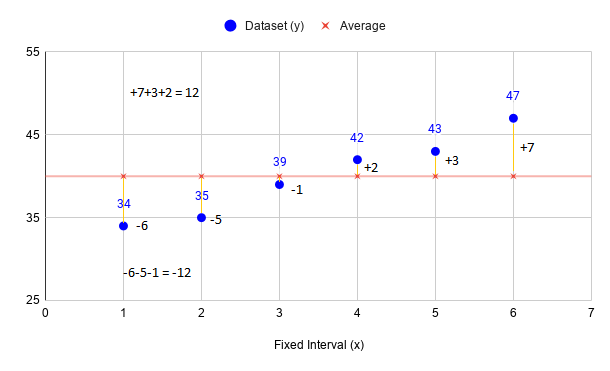Fig 2. Realize that distances are balanced! The sum of the distance above the line balances the sum of those below the line.

Now let’s squared the errors out and take the sum of them all:

Now, what if I move the Average Line (red) a bit up? 45 instead.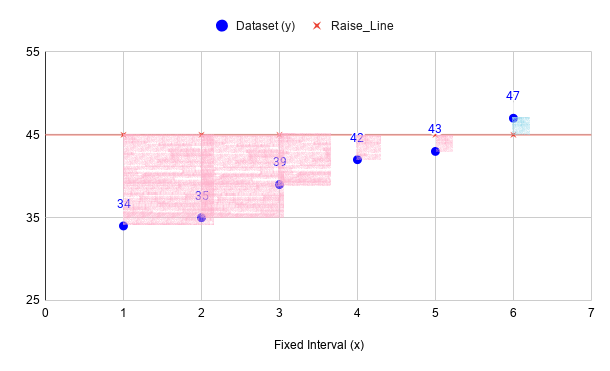Fig 3. Moving the line just a little drastically adding to the sum of the squares. Check it out!

Even if I shift that up by just a mere 5 units (45–40) by squaring the error that the effects drastically change 65 units (153–88).

That’s it! The goal of the regression is to find the line that best describes our data, by minimizing the Sum of Square Error (SEE).

Fortunately, we don’t have to rely on trial-and-error… actually you can now with GeoGebra: https://www.geogebra.org/m/B7JtA6Mg

We did with only one variable to calculate the mean but if you are dealing with two variables you’re not going to do that so directly.

We have to use algebra!

We need a system of the equation that can solve the line that best fits for two variables for regression (or even more for multiple regression…).

Recall that the equation for the line follows the formula:

`y = mx + bWhere: m is the slope of the line        b is where the line crosses the y-axis when x=0 (y-intercept) `

In linear regression, where we try to formulate the relationship between variables, the y = mx + b becomes: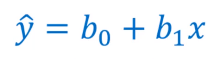Fig 4. Hat means predictions! A prediction is a forecast, but not only about the weather. Pre means “before” and diction has to do with talking. So a prediction is a statement about the future. It’s a guess, sometimes based on facts or evidence, but not always.

Our goal is to predict the value of the dependant variable (y) based on that independent variable (x).

How to derive b1 and b0?

Let’s get down to practice!

`A manager wants to find the relationship between the number of hours that a plant is operational in a week and weekly production.`

Here the independent variable x is hours of operation, and the dependent variable y is production volume.

The manager develops the following table:

`x -  y--    --34 - 10235 - 10939 - 13742 - 14843 - 15047 - 158production Hour (x) - Production Volume (y)`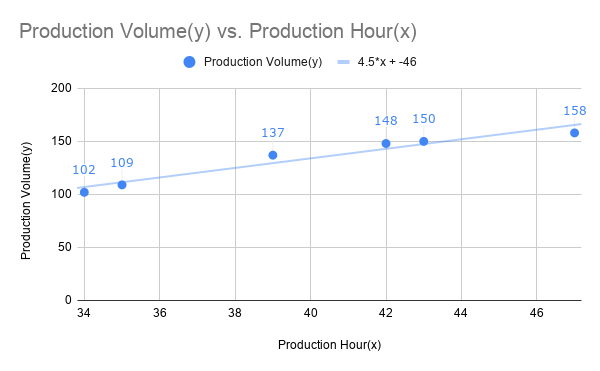Fig 6. This is our Regression Line equation: f(x) = 4.5x-46 that Google Calc gives me!

Here are all the calculations by hand (SSE, SSR SST, and R²)…

Now, what if the manager wants to produce 125 units per week, the plant should run for (confirm it all with Python below) how many hours?

`125 = 4,5x - 46x = 171/4.5 = 38 hours`

Now we can have a good estimate for how many hours per week that plant should be running (at least for what has been collected so far;).

But, there is Python\o/ 🎉

# Python

Below 10 Python solutions (you can download the complete Jupyter notebook from my GitHub Repo:)

00#PyEx — Python — Jupyter Notebook (VSC)

`Let's test Python in Jupyter Notebook (Visual Studio Code) and each of these Data Science & Statistic Libraries (scikit & statsmodels).If you haven't installed the Jupyter Notebook on VSC please see this post :)`

The solution, Type in each Jupyter cell:

`print("Hello")import pandas as pdimport seaborn as sns# We’ll be using Statsmodels since it has some nice characteristics # for linear modeling.# https://www.statsmodels.org/stable/index.htmlimport statsmodels.formula.api as smf# I am also using scikit-learn since it provides significantly more # useful functionality for machine learning.# https://scikit-learn.org/stable/from sklearn.linear_model import LinearRegressionfrom sklearn import metricsfrom sklearn.model_selection import train_test_splitimport numpy as np# allow plots to appear directly in the notebook%matplotlib inline# In terminal (Terminal>New Terminal) if error occurs, run:pip install pandaspip install seabornpip install statsmodelspip install sklearn`

`Download plant_prod_hourly.csv file from my GitHub Repo to load the data to be analyse hereafter(same directory as notebook file).`

The solution:

`# read data into a DataFramedata = pd.read_csv('plant_prod_hourly.csv')data.head()`

02#PyEx — Python —The shape of the DataFrame

`# print the shape of the DataFramedata.shape`

The solution:

`# shape of the DataFramedata.shape(6, 2)Meaning: There are 6 observations, and thus 2 variables in the dataset.`

03#PyEx — Python — Visualization

`# visualize the relationship between the features and the response `

The solution:

`using scatterplots# Create the default pairplotsns.pairplot(data)<seaborn.axisgrid.PairGrid at 0x1dab290ca20>`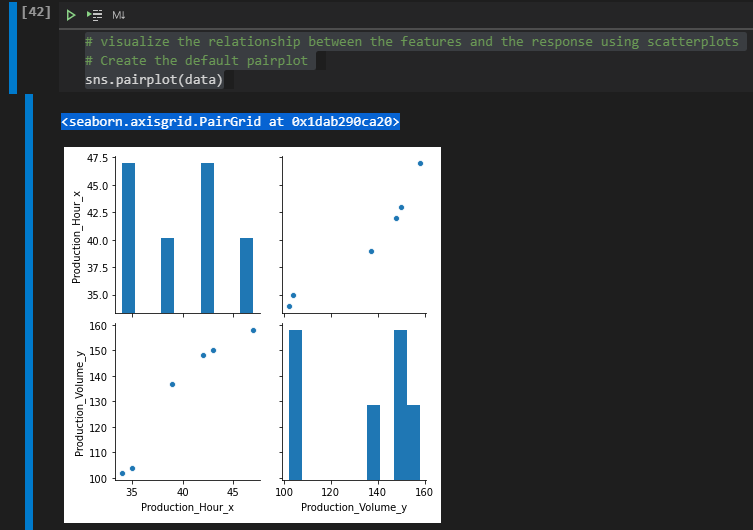Fig 8. Matriz plot for the initial checks out: there is a sign of a positive correlation between the variables!

04#PyEx — Python — Estimating Model Coefficients

`### SCIKIT-LEARN & SCIKIT-LEARN ###`

1ª solution: STATSMODELS library (lm1):

`# create X and y# create a fitted modellm1 = smf.ols(formula='Production_Volume_y ~ Production_Hour_x', data=data).fit()# print the coefficientslm1.paramsIntercept           -46.0Production_Hour_x     4.5dtype: float64`

2ª solution: SCIKIT-LEARN library (lm2):

`feature_cols = ['Production_Hour_x']X = data[feature_cols]y = data.Production_Volume_y# Initiate and fitlm2 = LinearRegression()lm2.fit(X, y)# print the coefficientsprint ("iNTERCEPT : ",lm2.intercept_)print ("CO-EFFICIENT : ",lm2.coef_)iNTERCEPT :  -45.99999999999994 CO-EFFICIENT :  [4.5]`

3ª solution: SCIPY library:

`from scipy.stats import linregressx=[34,35,39,42,43,47]y=[102,109,137,148,150,158]slope=round(linregress(x,y).slope,1)intercept=round(linregress(x,y).intercept,1)print(f'y={intercept} + {slope}x')y=-46.0 + 4.5x`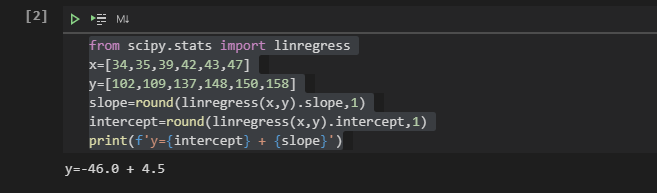Fig 9. Here I am using Jupyter Notebook. If you haven’t already, consider to init by this post:) → https://medium.com/jungletronics/python-jupiter-notebook-quick-start-with-vscode-916c43c10d9a

4ª solution: NUMPY & MATPLOTLIB library:

`import numpy as npimport matplotlib.pyplot as pltfrom scipy import statsx=np.array([34,35,39,42,43,47])y=np.array([102,109,137,148,150,158])a,b,correlation,p,error=stats.linregress(x,y)print('Regression line: y=%.2fx+%.2f'% (a,b))print('Correlation Coefficient: r=%.2f'% correlation)plt.plot(x,y,'o',label='Original data')f=a*x+bplt.plot(x,f,'r',label='Regression Line')plt.ylim(100, 180)plt.legend()plt.show()Regression line: y=4.50x+-46.00 Correlation Coefficient: r=0.97`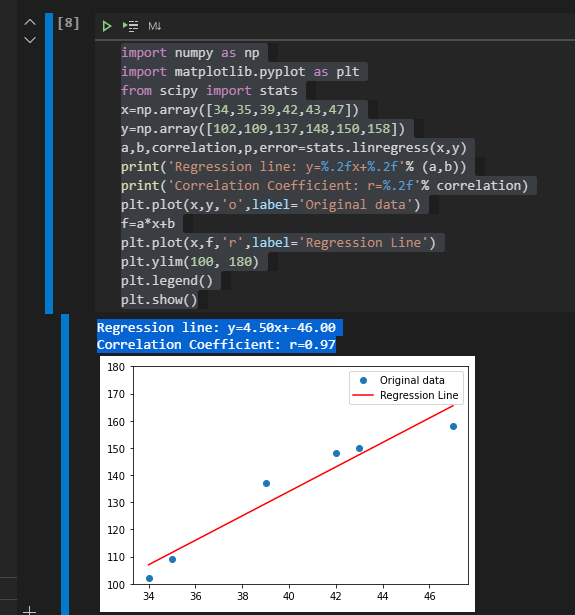Fig 10. As r=.97 (this gives us the Correlation Coefficient (R = 0.97)— see R² below), our model is very good at estimate our production!

05#PyEx — Python — Inferences

`### STATSMODELS #### you have to create a DataFrame since the Statsmodels formula # interface expects it`

The solution:

`X_new = pd.DataFrame({'Production_Hour_x': })# predict for a new observationlm1.predict(X_new)0    516.5dtype: float64# 125 hour of productions yield for 532 volume units; # manually calculate the prediction to confirm the above calculation :)-45.99999999999994 + 4.5*125516.5`

06#PyEx — Python — Predicting

`### SCIKIT-LEARN #### predict for a new observation`

The solution:

`lm2.predict([])array([125.])We would predict Production of 125 units in that market by working 38 hours.`

07#PyEx — Python — Plotting the Least Squares Line

`# Let’s plot the least squares line for Production versus Volume`

The solution:

`sns.pairplot(data, x_vars=['Production_Hour_x','Production_Volume_y'], y_vars='Production_Hour_x', size=7, aspect=0.7, kind='reg')<seaborn.axisgrid.PairGrid at 0x1dab5a75400>`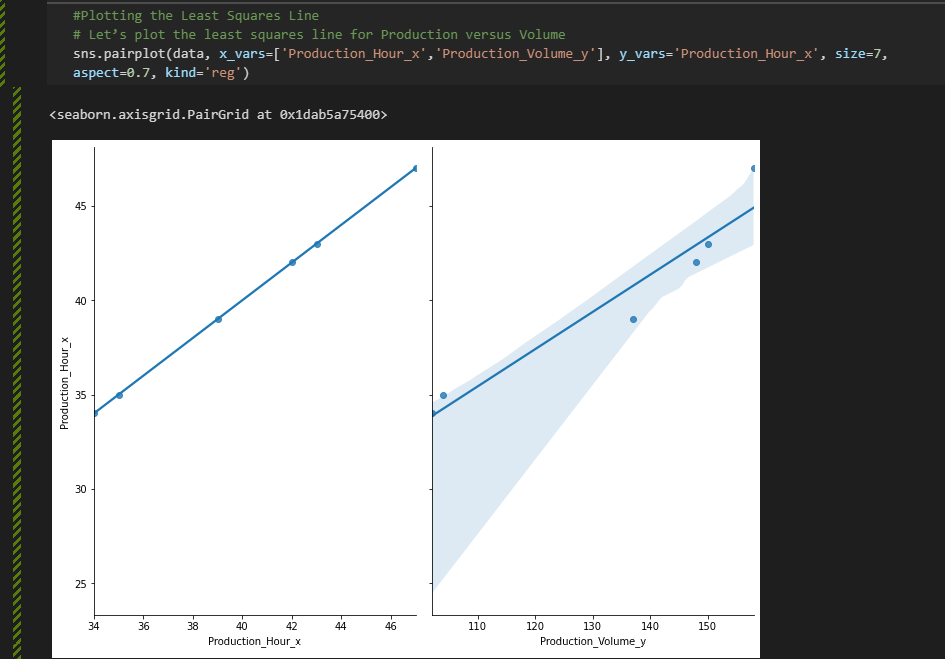Fig 11. Production x Volume - Area of uncertainty, Possible Values :/ Region

08#PyEx — Python — Hypothesis Testing and P-Value

`### STATSMODELS #### print the p-values for the model coefficients`

The solution:

`lm1.pvaluesIntercept            0.126734Production_Hour_x    0.001628dtype: float64`

Meaning:

Hypothesis Testing and p-values

Generally speaking, you start with a null hypothesis and an alternative hypothesis (that is opposite the null). Then, you check whether the data support rejecting the null hypothesis or failing to reject the null hypothesis.

(Note that “failing to reject” the null is not the same as “accepting” the null hypothesis. The alternative hypothesis may indeed be true, except that you just don’t have enough data to show that.)

As it relates to model coefficients, here is the conventional hypothesis test:

. null hypothesis: There is no relationship between Production and Plant Operating Hours (and thus β1β1 equals zero)

. alternative hypothesis: There is a relationship between Production and Plant Operating Hours (and thus β1β1 is not equal to zero)

A p-value of less than 0.05 is one way to decide whether there is likely a relationship between the feature and the response.

In this case, the p-value for Production_Hour_x is far less than 0.05 (Production_Hour_x 0.001628), and so we believe that there is a relationship between Production and Plant Operating Hours (see R and R² on Geogebra).

We generally don’t consider the p-value for the intercept.

09#PyEx — Python — R-squared — R² (STATSMODELS)

`### STATSMODELS ###`

The solution:

`# print the R-squared value for the modelprint("StatModel R-Square Value",lm1.rsquared)# print the R-squared value for the modelprint("StatModel R-Square Value",lm1.rsquared)StatModel R-Square Value 0.9348473566641846`

10#PyEx — Python — R-squared (SCIKIT-LEARN) & Summary (STATSMODELS)

`### SCIKIT-LEARN #### print the R-squared value for the model`

The Summary:

`print("SkLearn R-Square Value",lm2.score(X, y))SkLearn R-Square Value 0.9348473566641846import statsmodels.formula.api as smfresults = smf.ols('Production_Volume_y ~ Production_Hour_x', data=data).fit()print(results.summary())Table below`

Now, finally, to really drive GLM theory point home together with our statistic sample, what is this curly error term below (Ɛ)?

`Population Regression Fucntion:Y = βo + β1 X + Ɛ`

It is the ERROR of the Model! No model is perfect! Ɛ exists!

It is a sort of godly glowing line here for this very reason you can never know it exactly!

The theory is that it does exist…

Every population theoretical distance to that Population Regression Function that we can never calculate that curly error term but it does exist in theory.

So that’s completely different from the original error terms we were dealing with, with lowercase e, that’s the distance to our sample regression line and we can calculate we actually can find each individual error term so we can minimize the sum of those error terms squared whereas we can never know what those curly error terms are that just exists in theory and for a given sample the sum of those curly error terms aren’t necessary and going to be zero

See, statistics are not everything!

We must fly by visual approach; you are the pilot, but you must proceed by visual reference and clear of clouds to the airport. The pilot must at all times have either the airport or the preceding aircraft in sight; we must plotting our data beforehand to see the resulting graph (use pairplot from scikit lib, it is like a breeze!).

SEE THE THOUGHT OF Anscombe’s Quartet: why statistics is not enough (vid from YY Ahn).

And finally, that’s all!

I hope you can be as surprised just as I am surprised by the 💪Power of Python as well as Geogebra(see graph below)!

Be sure to watch the video linked below and subscribe to my YouTube channel to be kept informed of all the passion about Arduino, Rpi, PIC, Blender, Lego, Unity3d, Geogebra, and more!

See you in next PySeries!

Bye, for now, o/

# References & Credits

Thank you, Mr. Ricardo A. Deckmann Zanardini — You are an Awesome Teacher! o/; Text edited while I’m studying Computer Engineer at Escola Superior Politécnica Uninter🏋!

https://www.datasciencecentral.com/profiles/blogs/10-types-of-regressions-which-one-to-use

# Posts Related:

00Episode#PySeries — Python — Jupiter Notebook Quick Start with VSCode — How to Set your Win10 Environment to use Jupiter Notebook

01Episode#PySeries — Python — Python 4 Engineers — Exercises! An overview of the Opportunities Offered by Python in Engineering!

02Episode#PySeries — Python — Geogebra Plus Linear Programming- We’ll Create a Geogebra program to help us with our linear programming

03Episode#PySeries — Python — Python 4 Engineers — More Exercises! — Another Round to Make Sure that Python is Really Amazing!

04Episode#PySeries — Python — Linear Regressions — The Basics — How to Understand Linear Regression Once and For All! (this one)

05Episode#PySeries — Python — NumPy Init & Python Review — A Crash Python Review & Initialization at NumPy lib.

06Episode#PySeries — Python — NumPy Arrays & Jupyter Notebook — Arithmetic Operations, Indexing & Slicing, and Conditional Selection w/ np arrays.

07Episode#PySeries — Python — Pandas — Intro & Series — What it is? How to use it?

08Episode#PySeries — Python — Pandas DataFrames — The primary Pandas data structure! It is a dict-like container for Series objects

09Episode#PySeries — Python — Python 4 Engineers — Even More Exercises! — More Practicing Coding Questions in Python!

10Episode#PySeries — Python — Pandas — Hierarchical Index & Cross-section — Open your Colab notebook and here are the follow-up exercises!

11Episode#PySeries — Python — Pandas — Missing Data — Let’s Continue the Python Exercises — Filling & Dropping Missing Data

12Episode#PySeries — Python — Pandas — Group By — Grouping large amounts of data and compute operations on these groups

13Episode#PySeries — Python — Pandas — Merging, Joining & Concatenations — Facilities For Easily Combining Together Series or DataFrame

14Episode#PySeries — Python — Pandas — Pandas Dataframe Examples: Column Operations

15Episode#PySeries — Python — Python 4 Engineers — Keeping It In The Short-Term Memory — Test Yourself! Coding in Python, Again!

16Episode#PySeries — NumPy — NumPy Review, Again;) — Python Review Free Exercises

17Episode#PySeriesGenerators in Python — Python Review Free Hints

18Episode#PySeries — Pandas Review…Again;) — Python Review Free Exercise

19Episode#PySeriesMatlibPlot & Seaborn Python Libs — Reviewing theses Plotting & Statistics Packs

20Episode#PySeriesSeaborn Python Review — Reviewing theses Plotting & Statistics Packs

…make both calculations and graphs. Both sorts of output should be studied; each will contribute to understanding.

— F. J. Anscombe, 1973

--

--

--

## More from Jungletronics

J of Jungle + 3 Plats Arduino/RPi/Pic = J3

## The top 20 CO₂ polluters, visualized## Bye Pandas, Meet Koalas: Pandas APIs on Apache Spark (Ep. 4)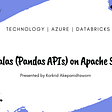## Articles Summary “Do you Know How to Scale Data in Big Data era?## Deep Learning Framework Power Scores 2018## Using Matplotlib and Pandas to Analyze a Region’s Economic Activity## Writing on Medium Got Me a Job in Data Analytics## Leam: Integrated, Interactive, and Extensible Text Data Analysis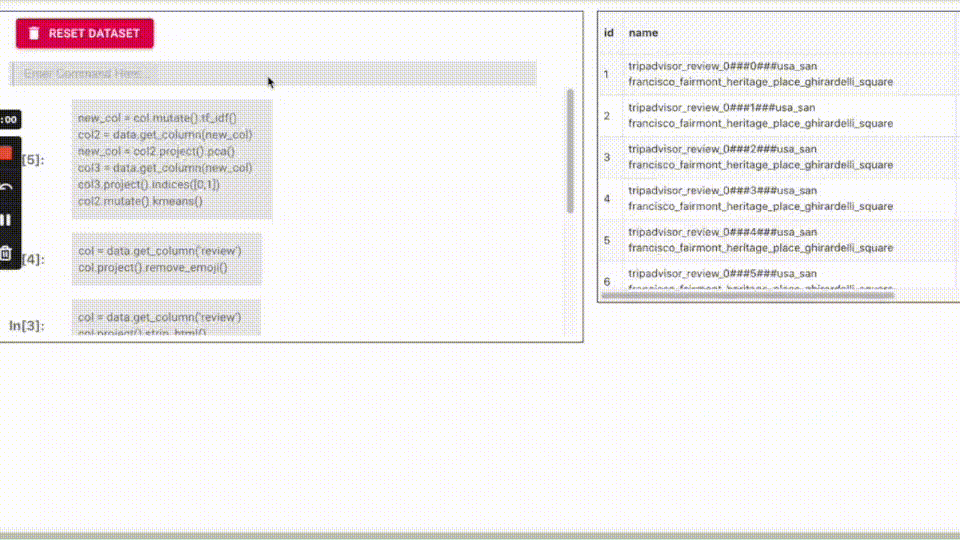## J3

Hi, Guys o/ I am J3! I am just a hobby-dev, playing around with Python, Django, Lego, Arduino, Raspy, PIC, AI… Welcome! Join us!

## String in Python